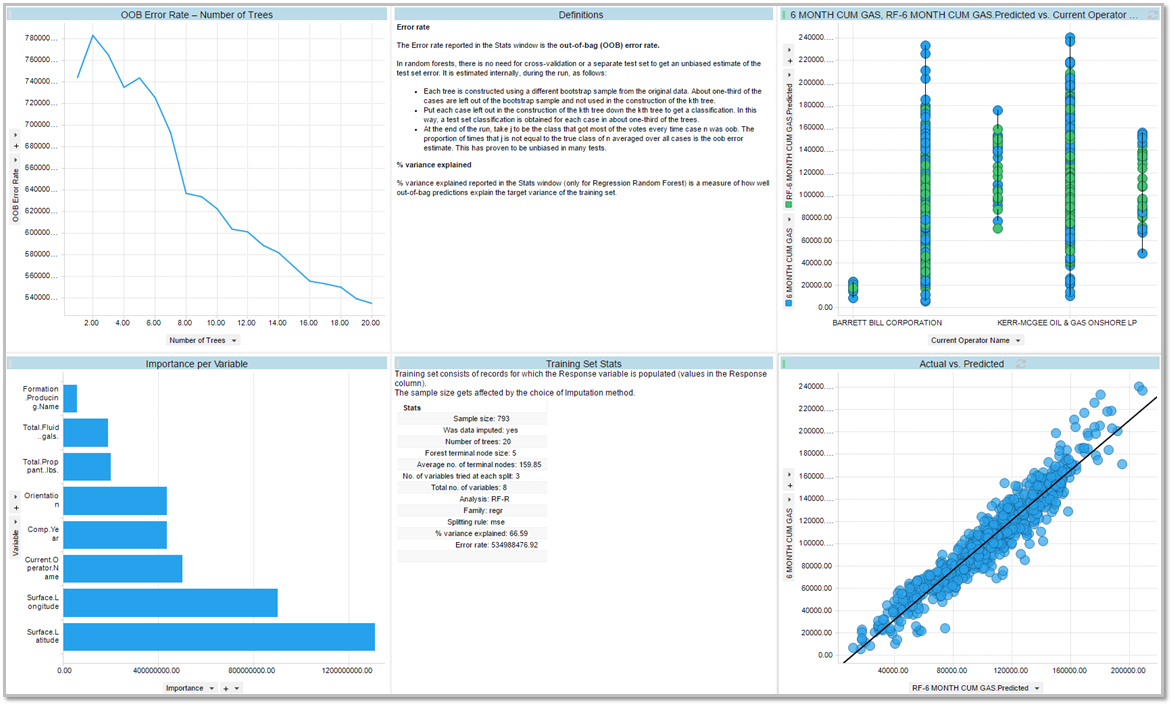## What is the Random Forest in Data Science Toolkit?

### Purpose

The Random Forest model is a form of multivariate analysis.  It uses an ensemble learning method for classification and regression.  The model constructs a "forest" of decision trees and generally does not overfit.

#### Outputs

• There are 6 outputs
• The OOB Error Rate - Number of Treelines chart shows the reduction in error as more tree are added to the ensemble.
• The Definition text area provides an explanation of error rate and % variance.
• The Training Set Stats table shows a print out of summary information on the model parameter and goodness of fit.
• The importance per Variable bar chart shows which variable in the model was the most important.
• The Actual vs Predicted scatter plots shows the values of the predicted response variable versus the actual value of the response variable in the dataset.
• The predicted response vs predictor column scatter plot shows how closely predicted the response column based on the unique values of all the variable in the model.

Note: The new prediction data will be added to a column in the original data table.
Example:Data Science Toolkit Random Forest User Guide: How to setup Random Forest

See Random Forest in action below

For additional information on RAI Data Science Toolkit documentation, click here.